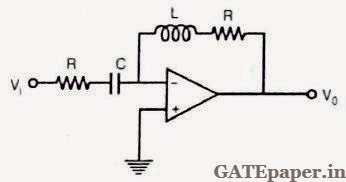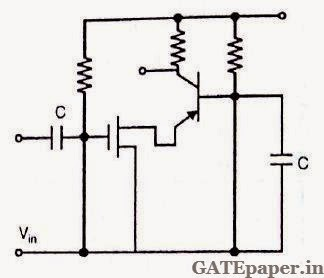### GATE 1988 ECE Analog Circuits (Analog Electronics) - Video Solutions

1. The OP-AMP shown in figure below is ideal and R = (L/C)1/2. The phase angle between Vo and Vi at ω = 1/(LC)1/2 isa. π/2
b. π
c. 3π/2
d. 2π

2. The quiescent collector current IC, of a transistor is increased by changing resistances. As a result
a. gm will not be effected
b. gm will decrease
c. gm will increase
d. gm will increase or decrease depending upon bias stability

3. Each transistor in the Darlington pair shown below has hFE = 100. The overall hFE of the composite transistor, neglecting the leakage currents isa. 10000
b. 10001
c. 10100
d. 10200

4. The amplifier circuit shown below uses a composite transistor of a MOSFET and BIPOLAR in cascade. All capacitances are large. gm of the MOSFET is 2 mA/V, and hfe of the BIPOLAR is 99. The overall Transconductance gm of the composite transistor isa. 198 mA/V
b. 9.9 mA/V
c. 4.95 mA/V
d. 1.98 mA/V

5. The transistor in the amplifier shown below has following parameters: hfe = 100, hie = 2 kΩ, hre = 0, hoe = 0.05 mhos. All capacitors are very large. The output impedance isa. 20 kΩ
b. 16 kΩ
c. 5 kΩ
d. 4 kΩ

#### 1 comment:

1.It it not wrong solution for question 3 since you have considered Ic2 as overall Ic. It should be Ic1+Ic2=Ic.in this case overall hfe= IC/Ib1 which is equal to hfe+hfe(1+hfe) =10200.please justify me

Name

Email *

Message *